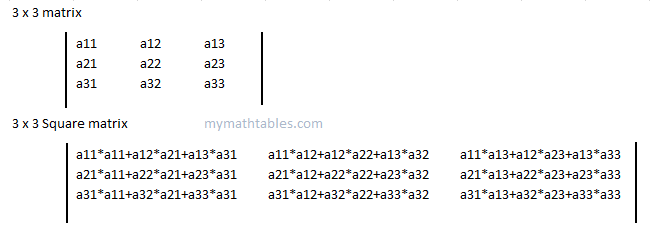# Squared Matrix Multiplication Three x Three (3*3)#3*3 Squared Matrix Formula & Calculation

An online Square Matrix calculation